# Trigonometry - Examples (with Solutions), Geometry, Quantitative Aptitude Notes | Study Quantitative Aptitude (Quant) - CAT

## CAT: Trigonometry - Examples (with Solutions), Geometry, Quantitative Aptitude Notes | Study Quantitative Aptitude (Quant) - CAT

The document Trigonometry - Examples (with Solutions), Geometry, Quantitative Aptitude Notes | Study Quantitative Aptitude (Quant) - CAT is a part of the CAT Course Quantitative Aptitude (Quant).
All you need of CAT at this link: CAT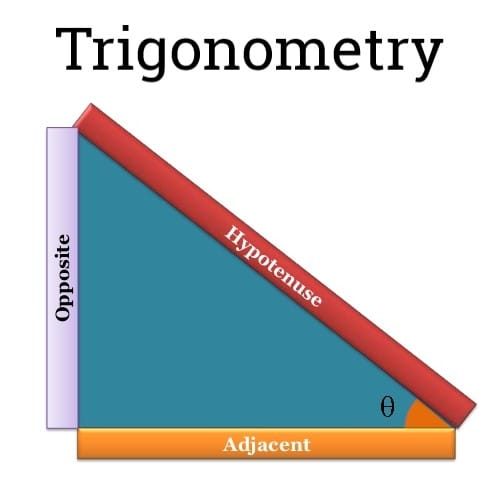Angles and their Relationship

• Angles are measured in many units’ viz. degrees, minutes, seconds, radians, gradients.
• Where 1 degree = 60 minutes, 1 minute = 60 seconds,
π radians = 180° = 200g
⇒ 1 radian = 180°/π and 1 degree = π/ 180 radians.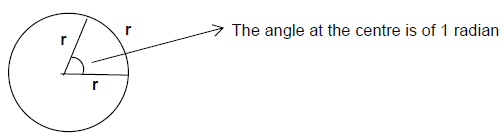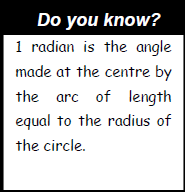Basic Trigonometric Ratios

• In a right triangle ABC, if θ be the angle between AC & BC.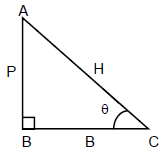• If θ is one of the angle other then right angle, then the side opposite to the angle is perpendicular (P) and the sides containing the angle are taken as Base ( B) and the hypotenuse (H). In this type of triangles, we can have six types of ratios. These ratios are called trigonometric ratios.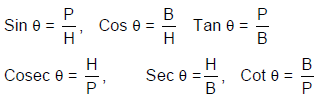Important Formulae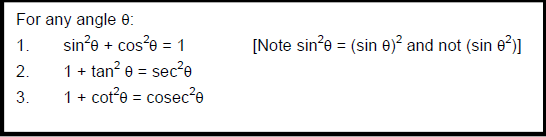Range of Values of Ratios

If 0 ≤ θ ≤ 360o, then the values for different trigonometric ratios will be as follows:

1. - 1 ≤ Sin θ ≤ 1
2. - 1 ≤ cos θ ≤ 1
3. - ∞ ≤ tan θ ≤ ∞
4. - ∞ ≤ cot θ ≤ ∞
5. - ∞ ≤ Sec θ ≤ -1    &       1 ≤ Sec θ ≤ ∞
6. - ∞ ≤ Cosec θ ≤ - 1     &      1 ≤ Cosec θ ≤ ∞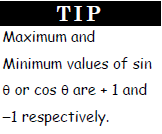Sign of Trigonometric Ratios

We divide the angle at a point (i.e. 360°) into 4 parts called quadrants. In the first quadrant all the trigonometric ratios are positive.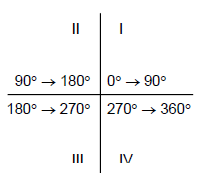Important Results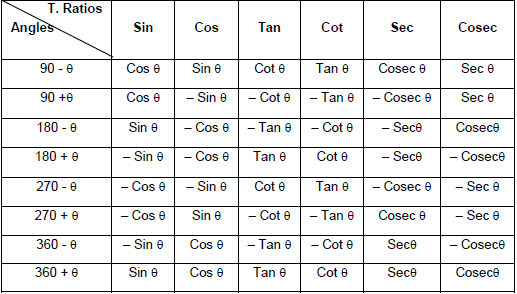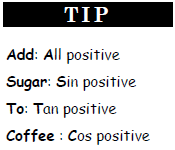Example.1 Simplify.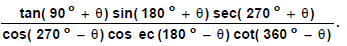Sol.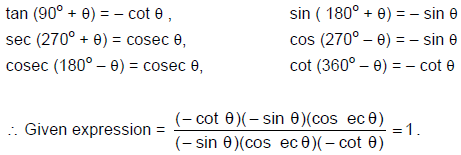Example.2 If cot A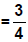, find the value of 3 cos A + 4 sin A, where A is in the first quadrant.

Sol.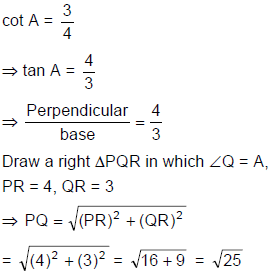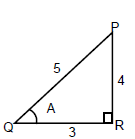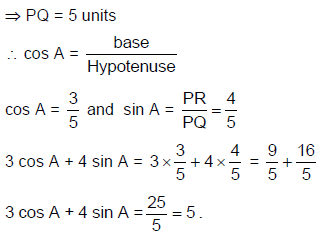Example.3 Find the value of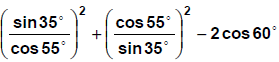Sol.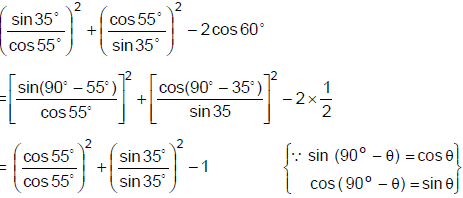= 1 + 1 - 1 = 1

Table: Values of trigonometric Ratio for some special angles.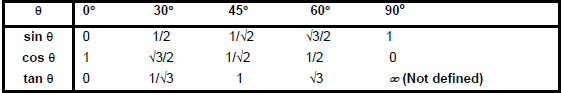Properties of Triangle

➢ Sine Rule

• In any triangle ABC if AB, BC, AC be represented by c, a, b respectively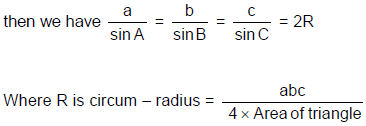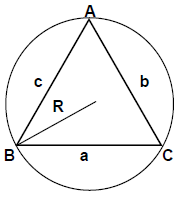➢ Cosine Rule

• In a triangle ABC of having sides of any size, we have the following rule: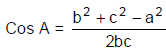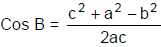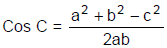➢  Area of Triangle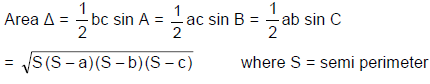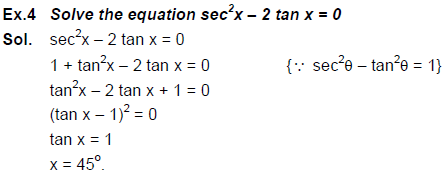The document Trigonometry - Examples (with Solutions), Geometry, Quantitative Aptitude Notes | Study Quantitative Aptitude (Quant) - CAT is a part of the CAT Course Quantitative Aptitude (Quant).
All you need of CAT at this link: CATUse Code STAYHOME200 and get INR 200 additional OFF

## Quantitative Aptitude (Quant)

155 videos|129 docs|131 tests

### How to Prepare for CAT

Read our guide to prepare for CAT which is created by Toppers & the best Teachers

Track your progress, build streaks, highlight & save important lessons and more!

,

,

,

,

,

,

,

,

,

,

,

,

,

,

,

,

,

,

,

,

,

,

,

,

,

,

,

;